## Selina Concise Mathematics Class 10 ICSE Solutions Chapter 20 Cylinder, Cone and Sphere (Surface Area and Volume) Ex 20F

These Solutions are part of Selina Concise Mathematics Class 10 ICSE Solutions. Here we have given Selina Concise Mathematics Class 10 ICSE Solutions Chapter 21 Trigonometrical Identities Ex 21F

Other Exercises

Question 1.
From a solid right circular cylinder with height 10 cm and radius of the base 6 cm, a right circular cone of the same height and same base is removed. Find the volume of the ramaining solid.
Solution:
Height of the cylinder (h) = 10 cm
and radius of base (r) = 6 cm.
∴ Volume of cylinder = πr2h
Height of cone = 10 cm
and radius of base of cone = 6 cm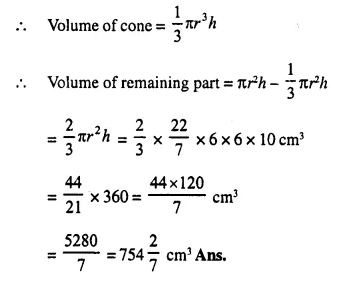Question 2.
From a solid cylinder whose height is 16 cm and radius is 12 cm, a conical cavity of height 8 cm and of base radius 6 cm is hollowed out Find the volume and total surface area of the remaining solid.
Solution:
Radius of solid cylinder (R) = 12 cm
and height (H) = 16 cm.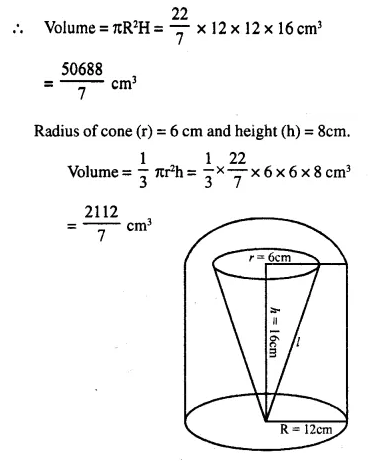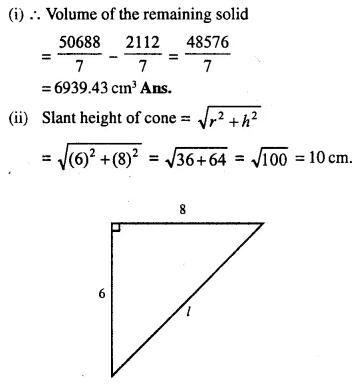Question 3.
A circus tent is cylindrical to a height of 4 m and conical above it. If its diameter is 105 m and its slant height is 80 m, calculate the total area of canvas required. Also, find the total cost of canvas used at Rs. 15 per metre if the width is 1.5 m.
Solution:
Radius of the cylindrical part of tent (r) = $$\frac { 105 }{ 2 }$$m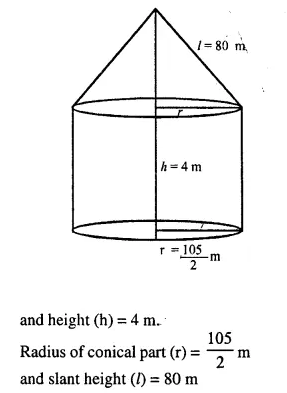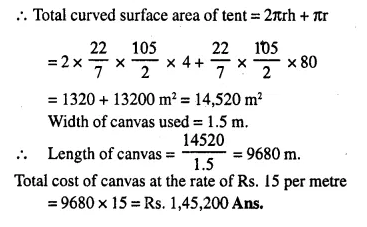Question 4.
A circus tent is cylindrical to a height of 8 m surmounted by a conical part. If total height of the tent is 13 m and the diameter of its base is 24 m; calculate:
(i) total surface area .of the tent,
(ii) area of canvas, required to make this tent allowing 10% of the canvas used for folds and stitching.
Solution:
Total height = 13 m
Diameter of base of the tent = 24 m
∴ Radius (r) = $$\frac { 24 }{ 2 }$$ = 12 m
Height of cylindrical part h1 = 8 m
and height of conical part (h2) = 13 – 8 = 5 mQuestion 5.
A cylindrical boiler, 2 m high, is 3.5 m in diameter. It has a hemi-spherical lid. Find the volume of its interior, including the part covered by the lid.
Solution:
Diameter of cylinderical boiler = 3.5 m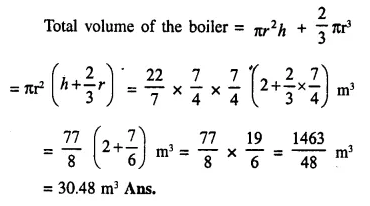Question 6.
A vessel is a hollow cylinder fitted with a hemispherical bottom of the same base. The depth of the cylindrical part is 4 $$\frac { 2 }{ 3 }$$ m and the diameter of hemisphere is 3.5 m. Calculate the capacity and the internal surface area of the vessel.
Solution: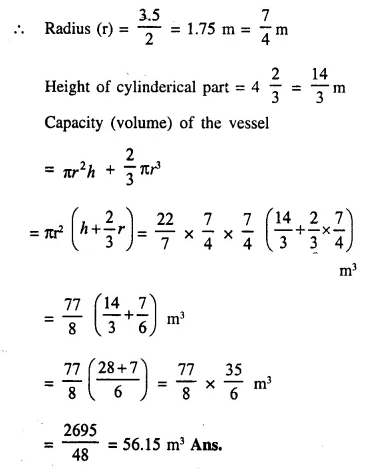Question 7.
A wooden toy is in the shape of a cone mounted on a cylinder as shown alongside.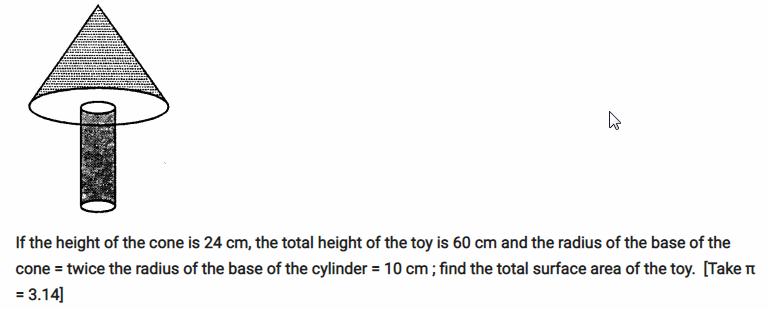If the height of the cone is 24 cm, the total height of the toy is 60 cm and the radius of the base of the cone = twice the radius of the base of the cylinder = 10 cm ; find the total surface area of the toy.  [Take π = 3.14]
Solution:
Height of the conical part (h1)= 24 cm
total height of the toy = 60 cm
∴ Height of cylinderical part (h) = 60-24 = 36 cm
Radius of the cone (r) = twice the radius of the cylinder = 10 cm
∴ Radius of cylinder (r1) = 5 cmQuestion 8.
A cylindrical container with diameter of base 42 cm contains sufficient water to sub­merge a rectangular solid of iron with dimensions 22 cm x 14 cm x 10.5 cm. Find the rise in level of the water when the solid is submerged.
Solution:
Diameter of cylinderical container = 42cm
∴  Radius (r) = $$\frac { 42 }{ 2 }$$ = 21 cm.
Dimension of a rectangular solid = 22 cm x 14cm x 10.5 cm
∴ Volume of solid
= 22 x 14 x 10.5 cm3        ….(i)
Let the height of water = h
∴ Volume of water in the container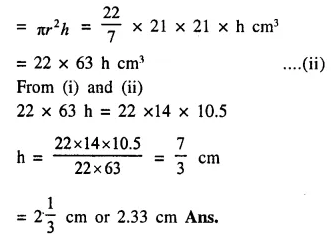Question 9.
Spherical marbles of diameter 1.4 cm are dropped into beaker containing some water and are fully submerged. The diameter of the beaker is 7 cm. Find how many marbles have been dropped in it if the water rises by 5.6 cm.
Solution:
Diameter of spherical marble = 1.4 cm.
∴ Radius = $$\frac { 1.4 }{ 2 }$$ = 0.7 cm.
Volume of one ball = $$\frac { 4 }{ 3 }$$ πr3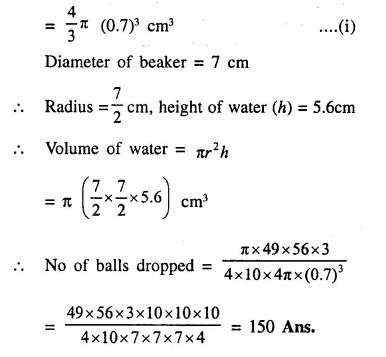Question 10.
The cross-section of a railway tunnel is a rectangle 6 m broad and 8 m high surmounted by a semi-circle as shown in the figure. The tun­nel is 35 m long. Find the cost of plastering the internal surface of the tunnel (excluding the floor) at the rate of Rs. 2.25 per m2.Solution:
and height = 8m
Length of tunnel = 35 m
Radius of semicircle = $$\frac { 6 }{ 2 }$$ = 3 m.
Circumference of semicircle = πr = $$\frac { 22 }{ 7 }$$ x 3 = $$\frac { 66 }{ 7 }$$ m
∴ Internal surface area of the tunnel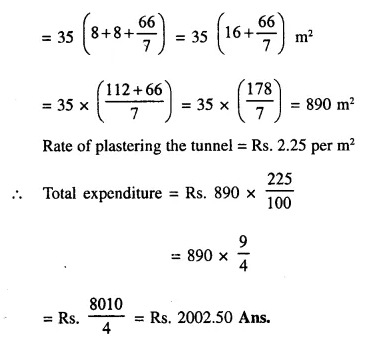Question 11.
The horizontal cross-section of a water tank is in the shape of a rectangle with semi-circle at one end, as shown in the following figure. The water is 2.4 metres deep in the tank. Calculate the volume of water in the tank in gallons.(Given : 1 gallon = 4.5 litres)
Solution:
Length = 21m
Depth of water = 2.4 m
∴ Radius of semicircle = $$\frac { 7 }{ 2 }$$ m.
Area of the cross section = Area of rectangle + area of semicircle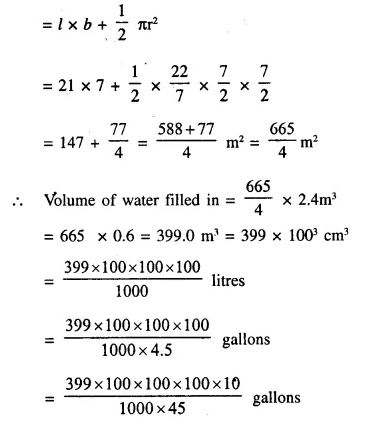Question 12.
The given figure shows the cross-section of a water channel consisting of a rectangle and a semi-circle. Assuming that the channel is always full, find the volume of water discharged through it in one minute if water is flowing at the rate of 20 cm per second. Give your answer in cubic metres correct to one place of decimal.Solution:
Rate of water flow = 20 cm/sec.
Period = 1 min. = 60 sec.
Radius of semi-circular part (r) = $$\frac { 21 }{ 2 }$$  cm
Height of channel (h) = 7 cm
Length of channel = 20 x 60 = 1200 cm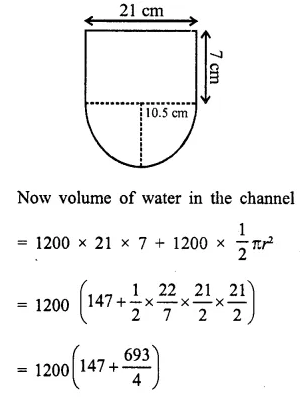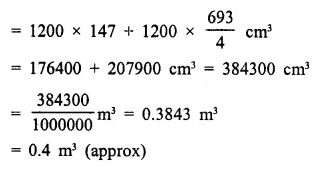Question 13.
An open cylindrical vessel of internal diameter 7 cm and height 8 cm stands on a horizontal table. Inside this is placed a solid metallic right circular cone, the diameter of whose base is 3 $$\frac { 1 }{ 2 }$$ cm and height 8 cm. Find the volume of water required to fill the vessel.
If this cone is replaced by another cone, whose height is 1 $$\frac { 3 }{ 4 }$$ cm and the radius of whose base is 2 cm, find the drop in the water level.  
Solution:
Diameter of cylinder = 7 cm
∴
Radius (R) = $$\frac { 7 }{ 2 }$$ cm
Height (h) = 8 cm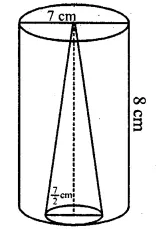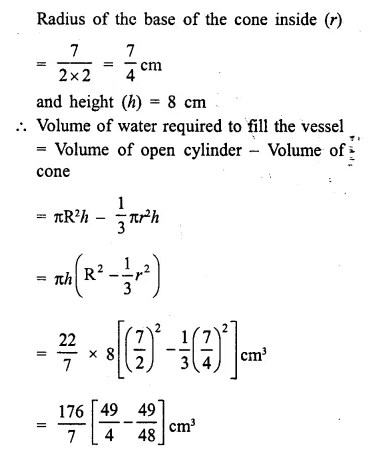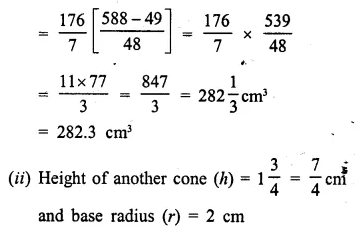Question 14.
A cylindrical can, whose base is horizontal and of radius 3.5 cm, contains sufficient water so that when a sphere is placed in the can, the water just covers the sphere. Given that the sphere just fits into the can, calculate:
(i) the total surface area of the can in contact with water when the sphere is in it;
(ii) the depth of water in the can before the sphere was put into the can. 
Solution:
Radius of the cylindrical can = 3.5 cm
∴  Radius of the sphere which fits in it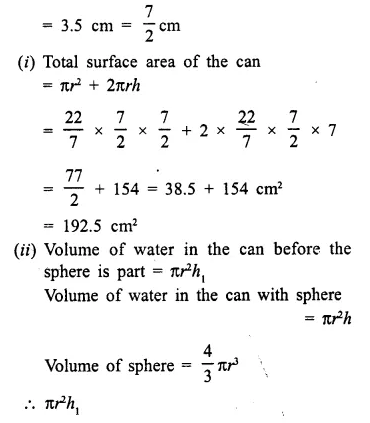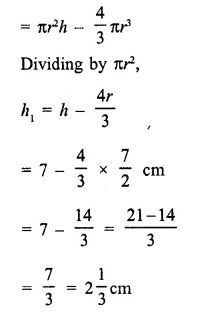Question 15.
A hollow cylinder has solid hemisphere inward at one end and on the other end it is closed with a flat circular plate. The height of water is 10 cm when flat circular surface is downward. Find the level’of water, when it is inverted upside down, common diameter is 7 cm and height of the cylinder is 20  cm.
Solution:
(i) Diameter of the cylinder = 7 cm
∴ Radius (r) = $$\frac { 7 }{ 2 }$$ cm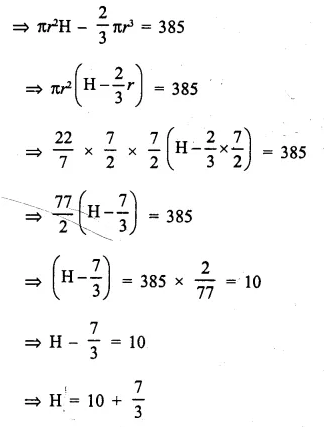Hope given Selina Concise Mathematics Class 10 ICSE Solutions Chapter 20 Cylinder, Cone and Sphere Ex 20F are helpful to complete your math homework.

If you have any doubts, please comment below. Learn Insta try to provide online math tutoring for you.